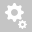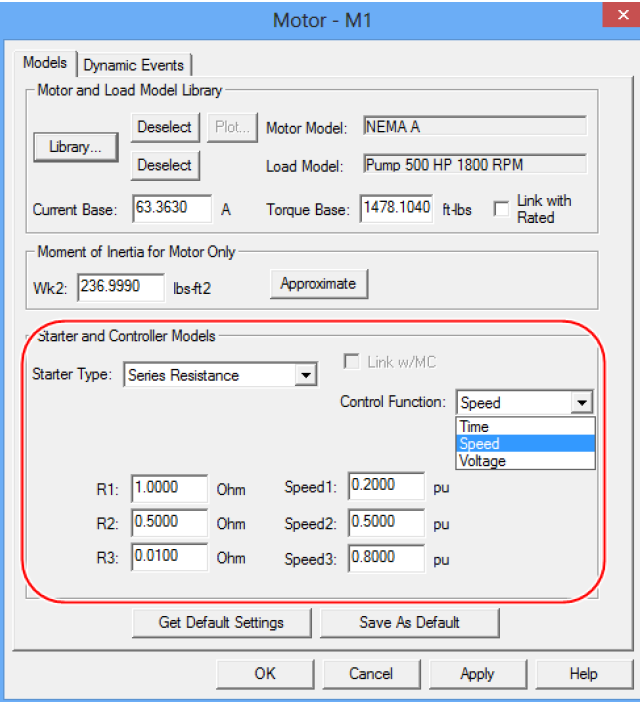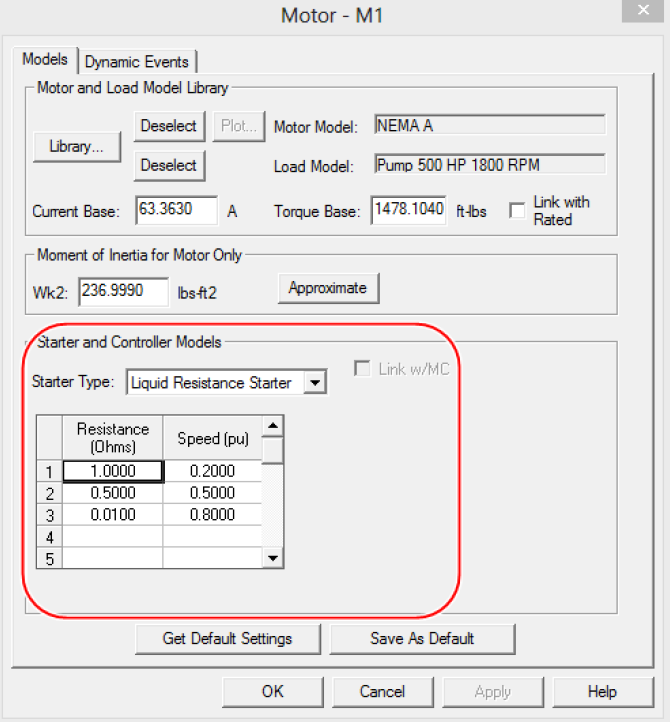##Recently viewed tickets• Sales
• Library Model Request
• Technical Support

# Difference between Series Resistance vs. Liquid Resistance Starter (General)

Question: In selecting motor starters/controllers for the TMS/ISIM studies, what is the difference between the Series Resistance and the Liquid Resistance Starters?

Answer:

Series Resistance - This controller reduces the amount of series impedance in up to three steps. These steps are controlled as a function of time, motor speed, or motor voltage. The control function time is related to the time the motor was switched on-line, not the time from the beginning of the Study. For example, if the motor switches on-line at three seconds, and you have entered T1 as one second, the reduction will occur at four seconds into the Study. If your controller only has two steps, leave the third box blank.Liquid Resistance Starter - This controller reduces the amount of series impedance in ohms up to several steps. These steps are controlled as a function of motor speed. You can enter the resistance (Ohms) to the corresponding Speed (pu) in the spreadsheet provided.Note: The Liquid Resistance Starter uses a linear interpolation continuous curve for resistance and speed (pu), while series Resistance used 3 discrete points.
That is for Liquid Resistance Starter, the corresponding resistance value that the software uses between Speed (pu) points is interpolated.
For Resistance Starter, the corresponding resistance value between Speed (pu) points is not interpolated. The higher impedance value is used between Speed (pu) points.